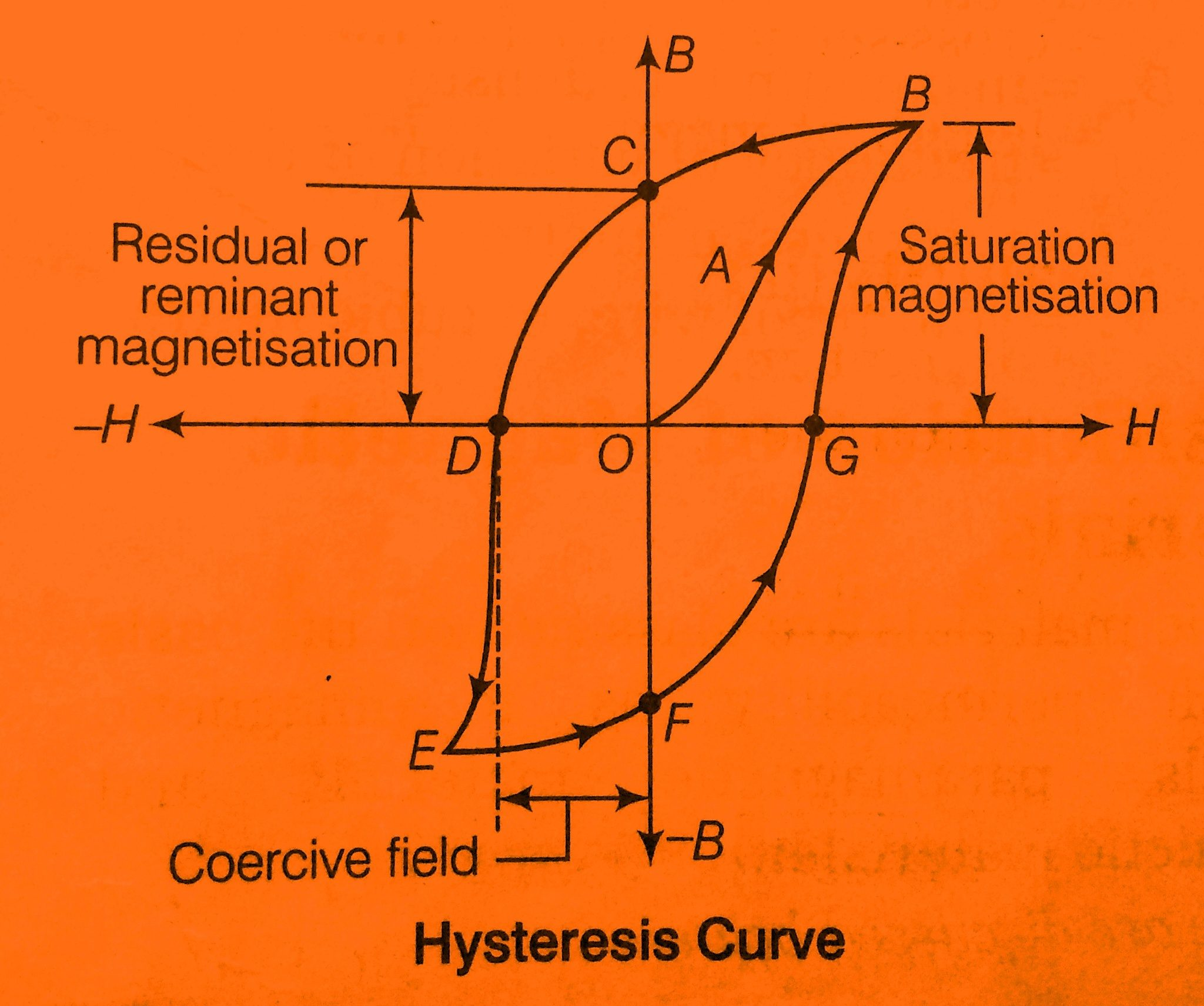# Hysteresis Loss and Formula of Hysteresis loss

## Hysteresis Loss and Magnetization curve

### MAGNETISATION CURVE OR B-H CURVE

The curve drawn giving the relationship between magnetic flux density (B) and the magnetizing force (H)is known as the magnetization curve or B-H curve.

When a magnetizing force is applied on a magnetic material, then the magnetizing flux density ‘B’ increases in direct proportion and like a straight line from the origin and then increase slowly as shown in the fig. but after reaching at a certain point the value of ‘B’ is constant i.e the part of the B-H curve becomes flat as shown in the figure below.

The point after which the value of magnetic flux density ‘B’ is constant then that is called the saturation point. And the flat part of the curve is known as the magnetic saturation.### MAGNETIC HYSTERESIS

When a magnetic material is magnetized first in one direction and then in another direction then it is found that the flux density ‘B’ in the magnetic material lags behind the applied magnetizing force ‘H’.

Hysteresis is defined as the phenomenon in which the magnetization lags behind the magnetizing force is called magnetic hysteresis.

Let us consider a solenoid wounded with N number of turns and current is passed through the solenoid then a magnetizing force ’H’ is produced directly proportional to the current through the solenoid.

Let the value of the magnetizing force is increased from zero to a certain maximum value and it then gradually decrease to zero.

For the corresponding values of the magnetizing force value of the magnetic flux density can be determined and the magnetization curve is drawn for increasing and decreasing values of the magnetizing force ‘H’ then it will be observed that the magnetization curve obtained for decreasing values of ‘H’ lies above that obtained for increasing values of H.

### RESIDUAL FLUX DENSITY (Br)/ RETENTIVITY

It is defined as the value of the flux stored in the magnetic material when the current in the magnetic material is reduced to zero then that value of the flux is known as the residual flux density or Retentivity.

### COERCIVE FORCE (Hc)/CO-ERCIVITY

The negative value of the magnetic field intensity given to a magnetic material to reduce the value of the residual flux density to zero is called a coercive force.

### HYSTERESIS LOSS

It is defined as the loss that occurred to neutralize the residual or remanent magnetism by the process of magnetization and demagnetization.

When a magnetic force is applied to a magnetic material then the magnetic material gets magnetized and the molecular magnets are lined up in a particular direction.

But if this magnetic force in magnetic gets reversed then the internal friction of the molecular magnets opposes the reversal of magnetism resulting in magnetic hysteresis. So to overcome the internal friction of molecular magnets a part of the magnetizing force is used.

The work done by the magnetic force against the internal friction of the molecular magnets produces heat. Here actually loss of energy occurs and that is wasted in the form of heat due to hysteresis is called hysteresis loss.

It occurs in all the magnetic parts of any electrical machine where there is a reversal of magnetization. This loss consequently increases the temperature of the electrical machine which is undesirable.

so during the construction of such part, a suitable magnetic material is selected. silicon steel is considered the most suitable for this as silicon steel material has minimum hysteresis loss.

This loss occurring in the magnetic material makes the magnetic material hot due to the conversion of electrical energy into heat energy hysteresis loss.

It directly depends on the frequency i.e as the frequency increases the value of the hysteresis loss increases.

 Hysteresis loss (WH)=ղ Bm1.6  f v

Here,

ղ = hysteresis constant

Bm=magnetic flux density (maximum value) in           weber/meter2

f=frequency (Hz)

V=volume of magnetic material (m3)

This can also be determined from the area of the hysteresis loop, if the shape of the loop is provided we can easily calculate the hysteresis loss by calculating the area of the given loop.

### Important points to remember about hysteresis loss

1.In the case of Dc this loss becomes zero.

2.It is directly proportional to the area of the B-H curve(width of the B-H curve).

3.In the case of the air-core transformer hysteresis loss is zero as the B-H curve is linear.

4.B-H curve for air is linear.

5.It is small for soft magnetic material as the width of the B-H curve is small.

6.It is more for hard magnetic material as the width of the B-H curve is more.

view more…